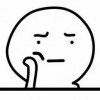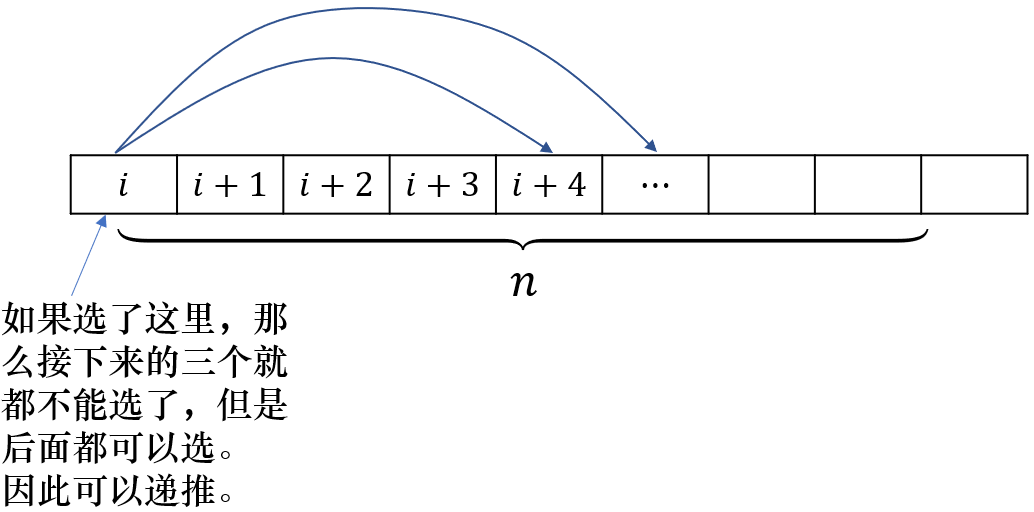2019-06-15 11:07:38

## 题解

c,t,r,l 分别表示喜欢唱、跳、rap、篮球的同学。## Code：

#include<cstdio>
#include<cstring>
#define p 998244353
#define ll long long
int Min(int x,int y){return x<y?x:y;}
ll qpow(ll x,ll y)
{
ll ans=1;
while(y)
{
if(y&1)
ans=ans*x%p;
x=x*x%p;
y>>=1;
}
return ans;
}
int f;
ll sum,func,inv;
int cnt;
int main()
{
int n;
scanf("%d",&n);
func=1;
f=1;
for(int i=1;i<=n;++i)
{
func[i]=func[i-1]*i%p;
f[i]=1;
for(int j=1;j<=i/4;++j)
{
sum[j-1]=(sum[j-1]+f[i-4][j-1])%p;
f[i][j]=sum[j-1];
}
}
inv[n]=qpow(func[n],p-2);
for(int i=n-1;i>=0;--i)
inv[i]=inv[i+1]*(i+1)%p;
int a,b,c,d;
scanf("%d%d%d%d",&a,&b,&c,&d);
int mn=a<b?(a<c?(a<d?a:d):(c<d?c:d)):(b<c?(b<d?b:d):(c<d?c:d)),ans=0;
//int mn=Min(Min(a,b),Min(c,d)),ans=0;
for(int i=0,tot=n;i<=mn&&i<=n/4;++i,--a,--b,--c,--d,tot-=4)
{
memset(cnt,0,sizeof(cnt));
long long op=0;
for(int A=0;A<=a;++A)
for(int B=0;B<=b;++B)
cnt[A+B]=(cnt[A+B]+inv[A]*inv[B])%p;
for(int C=0;C<=c;++C)
for(int D=0;D<=d&&C+D<=tot;++D)
op=(op+cnt[tot-C-D]*inv[C]%p*inv[D])%p;
op=op*func[tot]%p;
if(i&1)
ans=(ans+p-op*f[n][i]%p)%p;
else
ans=(ans+op*f[n][i]%p)%p;
}
printf("%d\n",ans);
return 0;
}
• star
首页